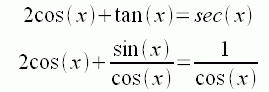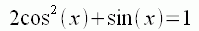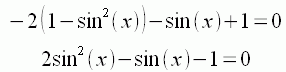SEARCH HOMEMath Central Quandaries & QueriesQuestion from tony, a student: list all real solutions of the equation that are in the interval [0,2π) 2cos(x) + tan(x) = sec(x)Hi Tony.

First convert this to sine and cosine:Then multiply by cos(x):Now use the identity sin2 (x) + cos2 (x) = 1 to change it all to a single trig function:And this is just a quadratic in sin(x). So you can use the quadratic formula if you wish, or try to factor it.

Can you finish the problem now?
Penny and Stephen La Rocque.Math Central is supported by the University of Regina and The Pacific Institute for the Mathematical Sciences.